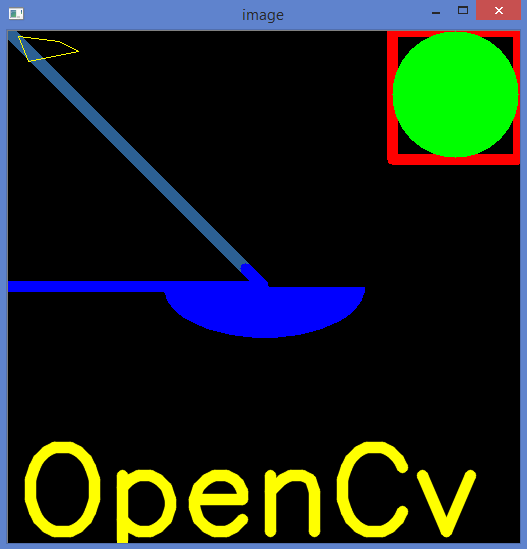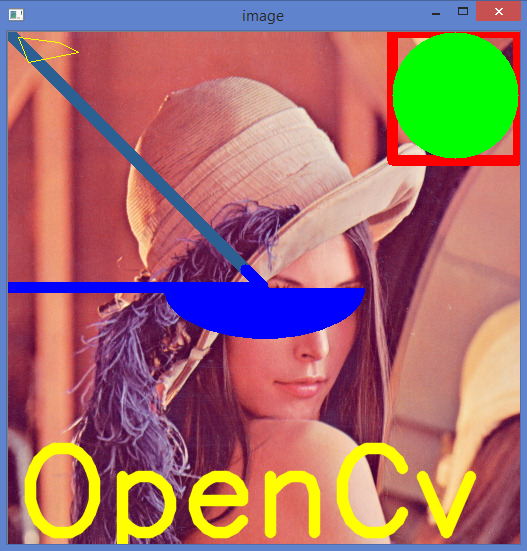# OpenCV Python Tutorial For Beginners – Draw geometric shapes on images using Python OpenCV

In this post on OpenCV Python Tutorial For Beginners, I am going to show How to Draw geometric shapes on images using Python OpenCV.
You will learn these functions : cv.line(), cv.circle() , cv.rectangle(), cv.ellipse(), cv.putText() etc.
cv2.line() : Used to draw line on an image.
cv2.rectangle() : Used to draw rectangle on an image.
cv2.circle() : Used to draw circle on an image.
cv2.putText() : Used to write text on image.

Result: